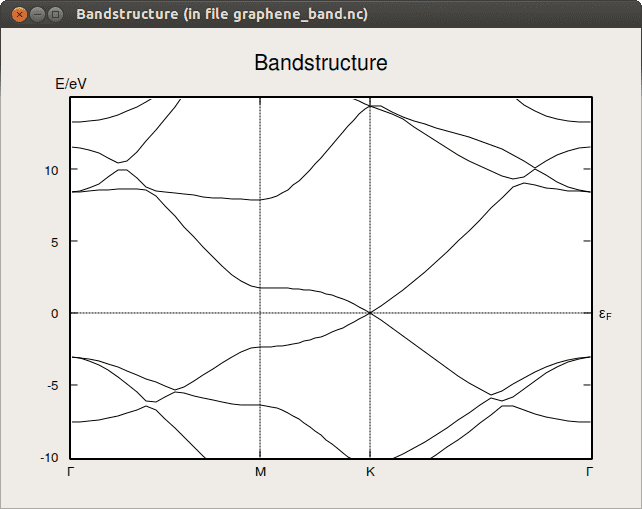# Band diagram, conductivity tensor

Hello!

Does anyone have an idea of how can I obtain information from a band diagram about the directions along which the system conducts best and worst ?

HAYAO
Gold Member
Do you mean Fermi surfaces?

I mean, I have a E vs k space diagram like this one, for example,(but imagine that I only have one line). I know that the velocity of the electron can be described as dE/dk. So that I can relate the different slopes of each part of my line with the velocity. At the same time, I know that the conductivity tensor depends on this velocity. Therefore, the higher the velocity the higher the conductivity. But now, how can I know the directions along which the system conducts best and worst (in the reciprocal space) and transform them to the real space?

(I hope I've explained myself well)

#### Attachments

Do you mean the resistivity along any direction? Something relevant would be :$$\mathbf{j}=\mathbf{\sigma}\cdot\mathbf{E}$$$$\mathbf{E}=\mathbf{\sigma}^{-1}\cdot\mathbf{j}=\rho\cdot\mathbf{j}$$$$\mathbf{j}_{\text{n}}=(-e)\int_{\text{occupied}}f(\epsilon_{\text{n}}(\mathbf{k}))\frac{d\mathbf{k}}{4\pi^3}\mathbf{v}_{\text{n}}(\mathbf{k})\to-ne\mathbf{v}_{\text{drift}}$$Are these thing what you're looking for?